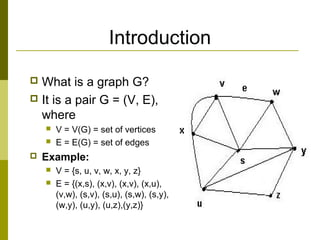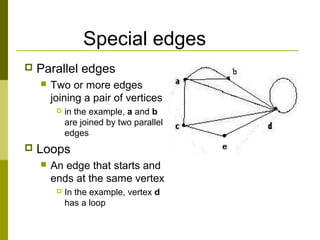Se está descargando tu SlideShare. ×

# Graph theory presentationCargando en…3
×

1 de 24
1 de 24

# Graph theory presentation

In mathematics, graph theory is the study of graphs, which are mathematical structures used to model pairwise relations between objects.Graph theory is also important in real life.

In mathematics, graph theory is the study of graphs, which are mathematical structures used to model pairwise relations between objects.Graph theory is also important in real life.

### Graph theory presentation

1. 1. Welcome to Discrete Mathematics PRESENTATION Topics: Graph Theory Submited by: Md: Aliul Kadir akib Daffodil International University Mail:aliulkadir@gmail.com
2. 2. Introduction  What is a graph G?  It is a pair G = (V, E), where  V = V(G) = set of vertices  E = E(G) = set of edges  Example:  V = {s, u, v, w, x, y, z}  E = {(x,s), (x,v), (x,v), (x,u), (v,w), (s,v), (s,u), (s,w), (s,y), (w,y), (u,y), (u,z),(y,z)}
3. 3. Special edges  Parallel edges  Two or more edges joining a pair of vertices  in the example, a and b are joined by two parallel edges  Loops  An edge that starts and ends at the same vertex  In the example, vertex d has a loop
4. 4. Special graphs  Simple graph  A graph without loops or parallel edges.  Weighted graph  A graph where each edge is assigned a numerical label or “weight”.
5. 5. Directed graphs (digraphs) G is a directed graph or digraph if each edge has been associated with an ordered pair of vertices, i.e. each edge has a direction
6. 6. Terminology – Undirected graphs  u and v are adjacent if {u, v} is an edge, e is called incident with u and v. u and v are called endpoints of {u, v}  Degree of Vertex (deg (v)): the number of edges incident on a vertex. A loop contributes twice to the degree (why?).  Pendant Vertex: deg (v) =1  Isolated Vertex: deg (v) = 0  Representation Example: For V = {u, v, w} , E = { {u, w}, {u, w}, (u, v) }, deg (u) = 2, deg (v) = 1, deg (w) = 1, deg (k) = 0, w and v are pendant , k is isolated
7. 7. Terminology – Directed graphs  For the edge (u, v), u is adjacent to v OR v is adjacent from u, u – Initial vertex, v – Terminal vertex  In-degree (deg- (u)): number of edges for which u is terminal vertex  Out-degree (deg+ (u)): number of edges for which u is initial vertex Note: A loop contributes 1 to both in-degree and out-degree (why?) Representation Example: For V = {u, v, w} , E = { (u, w), ( v, w), (u, v) }, deg- (u) = 0, deg+ (u) = 2, deg- (v) = 1, deg+ (v) = 1, and deg- (w) = 2, deg+ (u) = 0
8. 8. Theorems: Undirected Graphs Theorem 1 The Handshaking theorem: (why?) Every edge connects 2 vertices ∑∈ = Vv ve2
9. 9. Theorems: Undirected Graphs Theorem 2: An undirected graph has even number of vertices with odd degree even Voof =⇒ ⇒ ⇒ ∈⇒ +== ∑ ∑∑∑ ∈ ∈∈∈ 2 21 Vv 1, VvVuVv deg(v)termsecond evenalsoistermsecondHence 2e.issumsinceevenisinequalitylastthe ofsidehandrighton thetermslast twotheofsumThe even.isinequalitylasttheofsidehandrightin thefirst termThe Vfor vevenis(v)deg deg(v)deg(u)deg(v)2e verticesdegreeoddtorefersV2andverticesdegreeevenofsettheis1Pr
10. 10. Definitions – Graph Type
11. 11. Simple graphs – special cases  Wheels: Wn, obtained by adding additional vertex to Cn and connecting all vertices to this new vertex by new edges. Representation Example: W3, W4
12. 12. Complete graph K n  Let n > 3  The complete graph Kn is the graph with n vertices and every pair of vertices is joined by an edge.  The figure represents K5
13. 13. Bipartite graphs  A bipartite graph G is a graph such that  V(G) = V(G1) ∪ V(G2)  |V(G1)| = m, |V(G2)| = n  V(G1) ∩V(G2) = ∅  No edges exist between any two vertices in the same subset V(Gk), k = 1,2
14. 14. Complete bipartite graph Km,n  A bipartite graph is the complete bipartite graph Km,n if every vertex in V(G1) is joined to a vertex in V(G2) and conversely,  |V(G1)| = m  |V(G2)| = n
15. 15. Connected graphs  A graph is connected if every pair of vertices can be connected by a path  Each connected subgraph of a non- connected graph G is called a component of G
16. 16. Paths and cycles  A path of length n is a sequence of n + 1 vertices and n consecutive edges  A cycle is a path that begins and ends at the same vertex
17. 17. Euler cycles  An Euler cycle in a graph G is a simple cycle that passes through every edge of G only once.  The Konigsberg bridge problem:  Starting and ending at the same point, is it possible to cross all seven bridges just once and return to the starting point?  This problem can be represented by a graph  Edges represent bridges and each vertex represents a region.
18. 18. Degree of a vertex  The degree of a vertex v, denoted by δ(v), is the number of edges incident on v  Example:  δ(a) = 4, δ(b) = 3,  δ(c) = 4, δ(d) = 6,  δ(e) = 4, δ(f) = 4,  δ(g) = 3.
19. 19. Sum of the degrees of a graph Theorem : If G is a graph with m edges and n vertices v1, v2,…, vn, then n Σ δ(vi) = 2m i = 1 In particular, the sum of the degrees of all the vertices of a graph is even.
20. 20. Shortest Path Problems • Directed weighted graph. • Path length is sum of weights of edges on path. • The vertex at which the path begins is the source vertex. • The vertex at which the path ends is the destination vertex. 0 3 9 5 11 3 6 5 7 6 s t x y z 2 2 1 4 3
21. 21. Example 1 2 3 4 5 6 7 2 6 16 7 8 10 3 14 4 4 5 3 1 • A shorter path will cost only 11
22. 22. Representations of graphs  Adjacency matrix Rows and columns are labeled with ordered vertices write a 1 if there is an edge between the row vertex and the column vertex and 0 if no edge exists between them v w x y v 0 1 0 1 w 1 0 1 1 x 0 1 0 1 y 1 1 1 0
23. 23. Euler’s formula  If G is planar graph,  v = number of vertices  e = number of edges  f = number of faces, including the exterior face  Then: v – e + f = 2